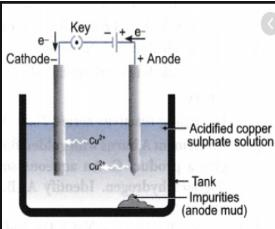Courses

# Physical Properties Of Metals -2

## 20 Questions MCQ Test Science Class 10 | Physical Properties Of Metals -2

Description
This mock test of Physical Properties Of Metals -2 for Class 10 helps you for every Class 10 entrance exam. This contains 20 Multiple Choice Questions for Class 10 Physical Properties Of Metals -2 (mcq) to study with solutions a complete question bank. The solved questions answers in this Physical Properties Of Metals -2 quiz give you a good mix of easy questions and tough questions. Class 10 students definitely take this Physical Properties Of Metals -2 exercise for a better result in the exam. You can find other Physical Properties Of Metals -2 extra questions, long questions & short questions for Class 10 on EduRev as well by searching above.
QUESTION: 1

### What happens when calcium is treated with water ? (i) It does not react with water. (ii) It reacts violently with water. (iii) It reacts less violently with water. (iv) Bubbles of hydrogen gas formed stick to the surface of calcium.

Solution:

Calcium reacts comparatively less violently with water and floats on the surface due to hydrogen gas sticking to the surface of the metal.

QUESTION: 2

### Which one of the following figures correctly describes the process of electrolytic refining ?

Solution:

Correct Answer :- c

Explanation:-Electrolytic refining is the process of refining impure metals by using electricity. In this process, impure metal is made the anode and a strip of pure metal is made the cathode. A solution of a soluble salt of the same metal is taken as the electrolyte. When an electric current is passed, metal ions from the electrolyte are deposited at the cathode as pure metal and the impure metal from the anode dissolves into the electrolyte in the form of ions. The impurities present in the impure metal gets collected below the anode. This is known as anode mud.

QUESTION: 3

### The ability of the metals to be drawn into thin wires is known as

Solution:

The ability of a metal to be drawn into wires is called ductility.

QUESTION: 4

Which of the following can undergo a chemical reaction ?

Solution:
QUESTION: 5

Which of the following metals exist in their native state in nature ?
(i) Cu
(ii) Au
(iii) Zn
(iv) Ag

Solution:

Au and Ag are unreactive metals and therefore exist in their native state.

QUESTION: 6

Although metals form basic oxides, which of the following metals form an amphoteric oxide ?

Solution:
QUESTION: 7

Galvanisation is a method of protecting iron from rusting by coating with a thin layer of

Solution:

Coating a metal with zinc is called galvanisation.

QUESTION: 8

The electronic configurations of three elements X, Y and Z are X —2, 8; Y — 2,8,7 and Z — 2, 8, 2. Which of the following is correct ?

Solution:

X = 2,8 = 10 : it is the electronic configuration of neon.
Y = 2,8,7 = 17 : it is the configuration of the halogen  family of chlorine atom which is not metal.
Z = 2,8,2 = 12 : it is the configuration of alkaline earth metals of magnesium atom which is metal.
Therefore, X is a noble gas, Y is a non Metal and Z is a metal.

QUESTION: 9

Stainless steel is very useful material for our life. In stainless steel, iron is mixed with

Solution:

Nickel and chromium are added to iron to make stainless steel.

QUESTION: 10

Reaction between X and Y, forms compound Z. X loses electron and Y gains electron. Which of the following properties is not shown by Z ?

Solution:
QUESTION: 11

Generally, non-metals are not lustrous. Which of the following non-metal is lustrous ?

Solution:

Iodine is a lustrous substance.

QUESTION: 12

Which among the following alloys contain mercury as one of its constituents ?

Solution:
QUESTION: 13

Which one of the following four metals would be displaced from the solution of its salts by other three metals ?

Solution:

Silver metal would be displaced from the solution of its salts by other three  metals because Ag is less reactive than Mg, Zn and Cu.

QUESTION: 14

Alloys are homogeneous mixtures of a metal with a metal or non-metal. Which among the following alloys contain non-metal as one of its constituents?

Solution:
QUESTION: 15

Which of the following are not ionic compounds ?
(i) KCl
(ii) HCl
(iii) CCl4
(iv) NaCl

Solution:

CCl4 is non-ionic, HCl becomes ionic only in water.

QUESTION: 16

An element A is soft and can be cut with a knife. This is very reactive to air and cannot be kept open in air. It reacts vigorously with water. Identify the element from the following

Solution:
QUESTION: 17

Electrical wires have a coating of an insulating material. The material, generally used is

Solution:

PVC is an abbreviated form of polyvinyl chloride. The electrical characteristics of PVC such as electrical insulating properties or dielectric constant are excellent and hence, are used as insulating materials.

QUESTION: 18

An electrolytic cell consists of
(i) positively charged cathode
(ii) negatively charged anode
(iii) positively charged anode
(iv) negatively charged cathode

Solution:
QUESTION: 19

Generally metals react with acids to give salt and hydrogen gas. Which of the following acids does not give hydrogen gas on reacting with metals (except Mn and Mg) ?

Solution:

Metals (except Mn and Mg) do not give hydrogen gas with nitric acid.

QUESTION: 20

2 mL each of concentrated HCl, HNO3 and a mixture of concentrated HCl and concentrated HNO3 in the ratio of 3 :1 were taken in test tubes labelled as A, B and C. A small piece of metal was put in each test tube. No change occurred in test tubes A and B but the metal got dissolved in test tube C respectively. The metal could be

Solution: